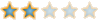# Week 1 Solutions## How hard were these week problems?0%
[ 0 ]0%
[ 0 ]33%
[ 1 ]67%
[ 2 ]0%
[ 0 ]

Total Votes : 3

##Week 1 Solutions

Dear users of this forum,

Congratulations on another great week of problems. Here are the official answers from Mr. Stephens.

Problem 1:
"The greatest digital sum date won't occur till almost the end of this century: 09/29/99, which has digital sum 38. "

Problem 2:
"Fifty miles is 5280 x 50 = 264,000 feet. So, a circle of radius 50 miles has approximately pi x (264,000)2 = (3.14)(69,696,000,000) = 218,845,440,000, or almost 219 billion, square feet of area. Since between 7 and 19 inches of snow fell in various areas, it's reasonable to assume that all areas received an average of (7 + 19) / 2 = 13 inches. And that layer of snow, we found, fell on 219 billion square feet of area. In total, that's 13 x (219 billion x 144) = 4.09 x 1014 cubic inches of snow. We were told that all of this snow weighed 5 billion tons, which is (5 x 109) x 2000 = 1.0 x 1013 pounds. Consequently, one cubic inch of snow weighs (1.0 x 1013) / 4.09 x 1014 = 0.024 pounds. Since there are 123 = 1728 cubic inches in a cubic foot, a cubic foot of this snow weighs 0.024 x 1728 = 41.5 pounds."

Problem 3:

"Every fourth year will be a leap year, except that three of the four years ending in double zero will not have leap days. Hence, every 400 years, there will be 400/4 - 3 = 97 leap days. From 2001 to 4000, there are 2000 years, so there will be 2000/400 x 97 = 485 leap days. As a fraction, that's 485/2000 = 97/400."

Problem 4:
"The same date in successive non-leap years occurs one day later in the week, so 2/28/1910 was a Monday and 2/28/1911 was a Tuesday. In a leap year, it's two days later, so 2/28/1912 was a Thursday. That means that 2/28/1913 was a Friday. Consequently, five days early, 23 February 1913, was the last Sunday in February that year. "

Problem 5:
"Use A, B and C to identify the number of votes received by each candidate. By using the information, B is greater than or equal to C/5, and is less than or equal to A/3. And, since B+C is greater than or equal to 600 and B is greater than or equal to C/5, 6C/5 is greater than or equal to 600. Therefore, C is greater than or equal to 500. By using the inequality above, B is greater than or equal to C/5, or 100. Since the least value of B is 100, the least value of A is 300 "

I hope you will soon be ready for next week's problems!Archis

##Re: Week 1 Solutions

Wait did you come up with those solutions archis or did mr. stephens send it to you?Steve
The Cool CubePosts : 85
Points : 4
Reputation : 4
Join date : 2008-10-07
Age : 22
Location : ...here##Re: Week 1 Solutions

Dear Steve,
Mr. Stephens sent it to me, as well as next week's problems. The setup is after a week alloted for Week 1, he emails me the Week 1 Solutions and Week 2 problems.

It follows like that for all instances.

Sincerely,
Archis

##Re: Week 1 Solutions

thats a good system!Steve
The Cool CubePosts : 85
Points : 4
Reputation : 4
Join date : 2008-10-07
Age : 22
Location : ...here##Re: Week 1 Solutions